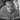# Rendering math equations with KaTeX

1 min read · tagged math, KaTeX

## Contents

gatsby-remark-katex adds math equation support to gatsby using remark-math and katex.

Surround your equation with $ to generate a math equation in inline mode. Example markdown: $a^2 + b^2 = c^2$ Example output: $a^2 + b^2 = c^2$ ## Math Equations in Display Mode Surround your equation with $$ and new-lines to generate a math equation in display mode. Example markdown: $$ a^2 + b^2 = c^2$\$

Example output:

$a^2 + b^2 = c^2$

Add Katex CSS to your template Katex’s CSS file is required to render the formulas correctly. Include the CSS file in your template (example)

require(katex/dist/katex.min.css)Daisy Buchanan is an attractive, if shallow and self-absorbed, young woman.